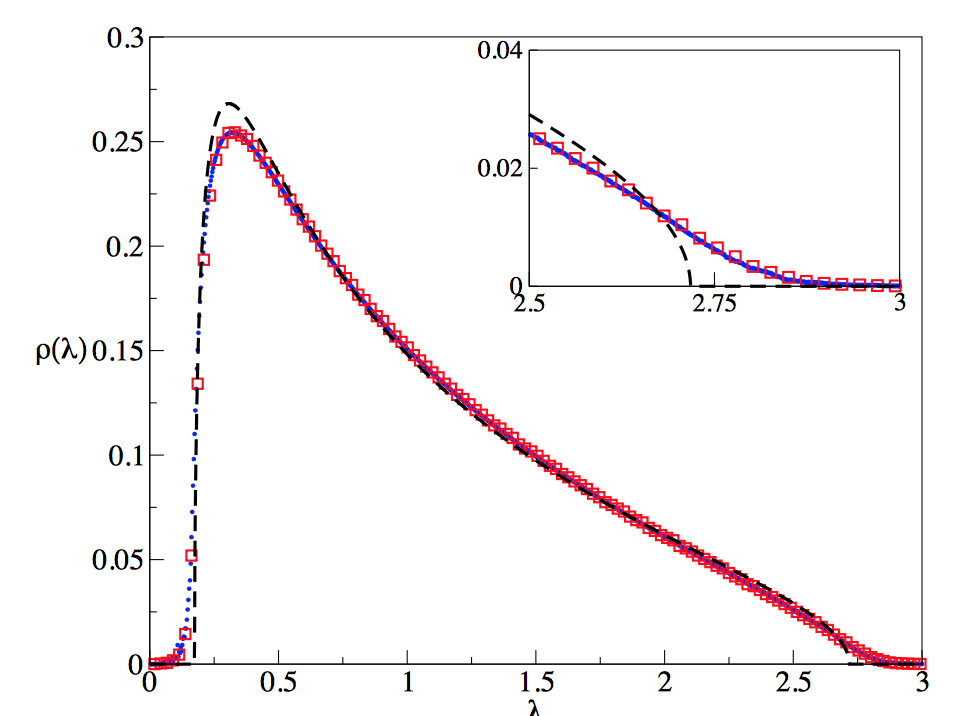# Singular values of sparse random real-valued matrix

I was wondering if anyone knew of any results regarding the limiting distribution of singular values for sparse random real-valued matrices?

Specifically, let $X$ be an $N\times M$ matrix with real-valued entries, with $X_{ij} = a_{ij}K_{ij}$, where the nonzero entries $K_{ij}\sim N(0, \sigma^2)$ i.i.d, and $a_{ij} = 1$ with probability $p$ and $0$ with probability $1-p$, i.i.d. We can assume here that $p = O(N^{-d})$ where $d > 0$.

What is known about analytically about the limiting distribution of the distribution of singular values of $X$ (or equivalently, the eigenvalue distribution of $X^TX$)?

There is a lot of literature on random matrix theory, and I figured there must be a result about this somewhere as this would be a variant of the Marchenko-Pastur law where the matrix has a well-defined sparsity. Pointers to references would be good too!

A general theoretical framework has been developed in Cavity Approach to the Spectral Density of Sparse Symmetric Random Matrices (2008), and an alternative approach is in Spectral Density of Sparse Sample Covariance Matrices (2006). Here is a plot for a binary $$\pm 1$$, rather than Gaussian, distribution of the nonzero entries ($$N=4000$$, $$\alpha=M/N=0.3$$, $$p=d/N=12/4000$$). The red points are a numerical simulation, the blue points are the "cavity theory" from the 2008 paper and the dashed curve is the "effective medium theory" from the 2006 paper. As you can see, both theories are of comparable quality, except near the spectral edge, where the former is better.• there is a closed form solution for the binary distribution in the 2006 paper (the "effective medium" theory); it is given on pages 14 and 15, involves solving a cubic equation; more generally, for the Gaussian distribution, you would need to solve a transcendental equation, which can only be done numerically; all of this is in the limit $N\rightarrow\infty$ for fixed $\alpha,p$, in which Marchenko-Pastur does not hold. Dec 19, 2016 at 7:16